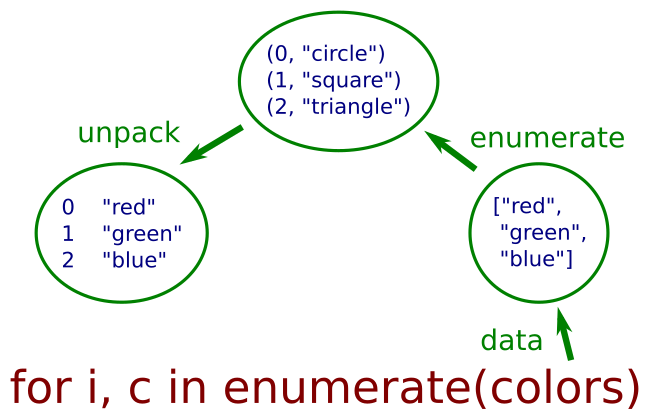# Using enumerate in a for loop

By Martin McBride, 2022-09-16
Tags: enumerate for loop
Categories: python language intermediate pythonEven though it is often best to avoid loop counters, there are times when they can be useful.

Fortunately, you don't have to forget everything you have learnt so far - you can use the enumerate function. This gives us access to the loop count within a Pythonic for loop.

## Enumerating a list

As a simple example, suppose we had a list of colours, and we wanted to print them out as a numbered list, like this:

0 red
1 green
2 blue


We might call this an enumerated listbecause each item has been assigned a number. How could we do this?

One way might be to go back to the old way of looping with a loop counter:

colors = ["red", "green", "blue"]

for i in range(len(colors)):
print(i, colors[i])


This works but is a bit messy. Alternatively, we could loop over the list without a loop counter, but maintain a separate count value:

i = 0
for c in colors:
print(i, c)
i += 1


Again, this works, but it is a bit clunky. Here is a better way:

for i, c in enumerate(colors):
print(i, c)


This time we use the built-in enumerate function to provide the enumeration. This function provides two loop variables:

• The first, i, is the incrementing count, starting from 0 by default.
• The second, c, is the current value out of the colors list.

We will see how this works in a little while.

## Using enumerate to provide a loop counter

We can use enumerate to provide a loop counter. In this example, we will print out a list, but we will mark one particular item as being selected:

selected = 1
for i, c in enumerate(colors):
if i == selected:
print("=>", c)
else:
print(c)


This code prints out all the items in the list, but prints the item that matches the selected value with a selected indicator. Item 1 (green) is selected, so it prints:

red
=> green
blue


By changing the value of selected we could mark a different item.

## How enumerate works

You might be wondering how enumerate creates two loop variables. It isn't anything too clever it is just uses tuple unpacking. To see how it works, take a look at this code:

for t in enumerate(colors):
print(t)


Here we are using enumerate with a single variable, t. This is what gets printed out:

(0, 'red')
(1, 'green')
(2, 'blue')


So enumerate creates a sequence of tuples. It converts:

["red", "green", "blue"]


Into:

[(0, 'red'), (1, 'green'), (2, 'blue')]


In general, it converts a sequence of values into a sequence of tuples, where the first value of each tuple is an incrementing counter. It doesn't actually create a list, it creates a lazy iterable, but the effect is the same.

If we wanted to extract the count and colour values we could do this:

for t in enumerate(colors):
i, c = t
print(i, c)


The loop creates tuples, as before, but we use tuple unpacking to get the values out of each tuple into separate variables i and c.

The next step is simply to move the unpacking to the for statement:

for i, c in enumerate(colors):
print(i, c)


This diagram illustrates the process:## Changing the start value

By default, the enumerate count values start at 0. This can be set to any value, via an optional second parameter. For example, this code starts the count at 1 instead of 0:

for i, c in enumerate(colors, 1):
print(i, c)


It prints:

1 red
2 green
3 blue


## Using enumerate with a list of tuples

We will look at a slightly different case next, where the original list contains tuples. Here is an example:

pairs = [("A", "X"), ("B", "Y"), ("C", "Z")]

for t in enumerate(pairs):
print(t)


This creates a sequence of nested tuples where the first element is the count, and the second element is the tuple from the pairs list:

(0, ('A', 'X'))
(1, ('B', 'Y'))
(2, ('C', 'Z'))


How can we unpack this nested tuple to get the three values? Unfortunately, this won't work, because it attempts to unpack the outer tuple into 3 values when it only contains 2 values:

for i, a, b in enumerate(pairs):
print(i, a, b)


We need to use this syntax to unpack the inner tuple:

for i, (a, b) in enumerate(pairs):
print(i, a, b)


This gives the expected result:

0 A X
1 B Y
2 C Z


## Using enumerate with zip

The zip function can be used with a for loop to loop over two lists in one loop (see the linked article for more details). Here is an example:

colors = ["red", "green", "blue"]
shapes = ["circle", "square", "triangle"]

for c, s in zip(colors, shapes):
print(c, s)


The zip function works in a similar way to enumerate. It combines two lists into a list of tuples:

[('red', 'circle'), ('green', 'square'), ('blue', 'triangle')]


These are then unpacked in the for statement into c and s values, giving the printed result:

red circle
green square
blue triangle


What if we want to enumerate this zipped data, and access all the values in the loop? Well, we can use the technique we used previously to enumerate a list of tuples, like this:

for i, (c, s) in enumerate(zip(colors, shapes)):
print(i, c, s)


This gives us:

0 red circle
1 green square
2 blue triangle


## Other useful techniques

List comprehension are another useful way to process iterables, especially if you need the result in the form of a list.

The itertools module contains several useful variants of zip and similar functions.